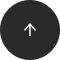Standard SQL

# How to Floor Numbers in SQL

FLOOR

#### Problem:

You want to round a number down to the nearest integer.

#### Example:

Our database has a table named employee with data in the following columns: `id`, `first_name`, `last_name`, and `hours_worked` (for the current month).

idfirst_namelast_namehours_worked
1AlanWatson95
2LisaBlack225
3LauraWilliams104

Let’s show the first and last name of each employee along with the number of days they worked. Since the table only shows the number of hours worked and not the days, we have to compute this value ourselves.

For this problem, we’ll assume that each employee has a standard eight-hour workday with no irregularities. Therefore, the number of days worked is the number of hours divided by eight.

#### Solution:

```SELECT last_name, first_name, FLOOR(hours_worked/8) AS days_worked
FROM employee;
```

The query returns the first and last name of each employee and the number of days they worked this month.

last_namefirst_namedays_worked
WatsonAlan11
BlackLisa28
WilliamsLaura13

#### Discussion:

Flooring is a simple mathematical operation that takes a number and rounds it down to the nearest integer. For example, the floor of 5 is 5 itself, while the floor of 5.1 is also 5. As another example, the floor of 4.9 is 4.

In SQL, we use the FLOOR function. It takes the number to round and returns the nearest integer that’s less than or equal to it. In our example, the number of days worked has to be an integer, not a floating-point number. Thus, we used (`FLOOR(hours_worked/8)`).

#### Recommended articles: